G-fibration

fibre bundle with a structure group

A generalization of the concept of the direct product of two topological spaces.

Letbe a topological group andan effective right-space, i.e. a topological space with a given right action ofsuch that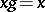for some,, implies. Letbe the subset of those pairs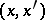for which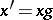for some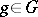, let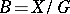be the orbit space, and let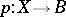be the mapping sending each point to its orbit. If the mapping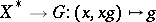is continuous, then the tuple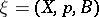is called a principal fibre bundle with structure group.

Letbe a left-space. The topological space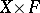admits a right action ofby,. The compositioninduces a mapping: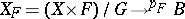(whereis the orbit space ofunder the action of). The quadruple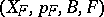is called a fibre bundle with structure group associated with the principal fibre bundle, and the quadruple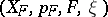is a fibre bundle with fibre, baseand structure group. Thus, a principal fibre bundle with a given structure group is a part of the structure of any fibre bundle with (that) structure group, and it uniquely determines the fibre bundle for any left-space.

If,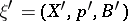are two principal fibre bundles with structure group, then a morphismis a mapping of-spaces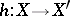.induces a mapping. A principal fibre bundle with structure group is called trivial is it is isomorphic to a fibre bundle of the following type: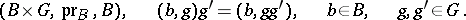Let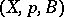be a principal fibre bundle and let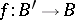be a continuous mapping of an arbitrary topological space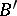into. Let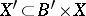be the subset of pairsfor which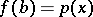. The projection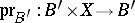induces a mapping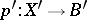. The space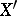has the natural structure of a right-space, and the tripleis a principal fibre bundle; it is induced byand is called an induced fibre bundle. Ifis the inclusion mapping of a subspace, then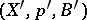is called the restriction ofover the subspace.

A principal fibre bundle with structure group is called locally trivial if its restriction to some neighbourhood of any point of the baseis trivial. For a wide class of cases, the requirement of local triviality is unnecessary (e.g. ifis a compact Lie group anda smooth-manifold). Hence, the term "fibre bundle" with structure group is often used in the sense of a locally trivial fibre bundle (or fibration).

Let,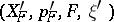be a pair of fibre bundles with the same structure group and the same-space as fibre. Given a morphismof principal fibre bundles, the mapping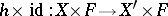induces a continuous mapping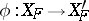, and the pair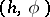is called a morphism of fibre bundles with structure group,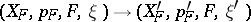.

A locally trivial fibre bundleadmits the following characterization, which gives rise to another (also generally accepted) definition of a fibre bundle with structure group. Let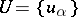be an open covering of the basesuch that the restriction ofto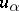is trivial for all. The choice of trivializations and their equality on the intersectionsleads to continuous functions (called transfer functions). On the intersection of three neighbourhoods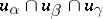one has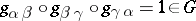, while the choice of other trivializations over every neighbourhood leads to new functions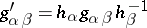. In this way, the functions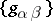form a one-dimensional Aleksandrov–Čech cocycle with coefficients in the sheaf of germs of-valued functions (the coefficients are non-Abelian), and a locally trivial fibre bundle determines this cocycle up to a coboundary.